## Estimation of in-situ water content, void ratio, dry unit weight and porosity using CPT for saturated sands

 Author(s) Nick Barounis, Johnny Philpot Published 24 November 2017 Collection NZGS Symposium Link N/A Compilation 20th NZGS Symposium
Estimation of in-situ water content, void ratio, dry unit weight and porosity using CPT for saturated sands

# Abstract

The CPT is used extensively for site characterization, soil profiling, determination of groundwater conditions and the estimation of geotechnical parameters. The geotechnical parameters that can be estimated by using the CPT include bulk unit weight, shear strength and stiffness, among many others. Best practice suggests the CPT is to be used in combination with laboratory testing, when the budget and timeframes allow for such testing to be undertaken. For typical structures of Importance Level 2, such testing is not undertaken due to a restricted budget. Typical soil classification tests, such as water content, bulk unit weight, sieve analysis and plasticity index, are not commonly performed for such projects. However, such tests are considered to be essential for the geotechnical engineer, who typically needs to adopt a number of parameters, relying solely on CPT information. Important parameters, that are often overlooked, are the water content, void ratio, dry unit weight and porosity. These parameters are important for characterizing the soil behavior, in both static and dynamic conditions.

This paper proposes a methodology for estimating the in-situ water content, void ratio, dry unit weight and porosity from CPT for saturated sandy soils. The methodology involves published work on estimating unit weight from CPT by Robertson (2010), combined with concepts of fundamental soil mechanics. The proposed methodology is applied on a predominantly sandy site in Christchurch, New Zealand and compared against laboratory results for the same site. Comments and conclusions are made on the usefulness and applicability of the proposed methodology along with recommendations for future applications.

# 1. INTRODUCTION

The CPT is a well-established strain-controlled undrained failure test that is typically performed by pushing a 35.7 mm diameter penetrometer, with a conical tip and an apex angle of 60°, vertically into the ground at a penetration rate of approximately 20 mm/s. The tip resistance stress of the cone qc and the sleeve friction resistance fs (both in MPa), are recorded versus the testing depth. Typically, qc is recorded against an incremental penetration of 10 mm. Other cone sizes and geometries are also available for pushing through dense gravels and deep soils.

The CPT is used extensively for delineating soil stratigraphy and estimating geotechnical parameters including bulk unit weight, relative density, cohesion, angle of friction and shear modulus (Robertson, 2015) for a wide range of soils. The correlations for geotechnical parameters from CPT are semi-empirical and vary in both reliability and applicability (Robertson, 2015). CPT is also a well-established test for evaluating the liquefaction potential (Robertson, 2015).

This paper presents an alternative method for estimating water content, void ratio, dry unit weight and porosity based on the well-established correlation by Robertson (2010). Based on this correlation, the bulk unit weight (γ) of each soil layer is estimated depending on the recorded qt (cone resistance corrected for pore water effects) and fs.

The correlation for the bulk unit weight is expressed by the following equation (Robertson, 2010):

w = 0.27 [log Rf] + 0.36 [log(qt/pa)] +1.236 (1)

Rf = friction ratio = (fs/qt) 100 %

w = unit weight of water, in same units as (kN/m3)

pa = atmospheric pressure, in same units as qt (MPa or kPa)

Prediction of the soil behaviour is achieved by means of the normalised Soil Behaviour Type (SBTn) (Robertson, 2015) for soils ranging from clays and silts to sands and gravels. Thus, a soil profile to the final testing depth can be inferred, accompanied by the corresponding unit weight. This procedure enables the geotechnical engineer to produce a continuous estimate of the total overburden stress. By delineating the location of the groundwater table, effective overburden stress can be also estimated.

In the absence of laboratory test results for any given project, the water content, void ratio, dry unit weight and porosity are four important parameters that are usually overlooked. These parameters are important for characterizing the soil behavior, in both static and dynamic conditions.

In this paper, a simple methodology is proposed for estimating the in-situ water content, void ratio, dry unit weight and porosity from CPT. The theoretical background, limitations and advantages of the proposed methodology are discussed in the next paragraphs.

# 2. THEORETICAL BACKGROUND OF THE PROPOSED METHOD

## 2.1 Soil phase relationships and limitations

The proposed method has the following important limitations:

• It is only applicable for inorganic soils: clays, silts, sands, gravels and their mixtures, for which the Gs values can be reliably assumed, or determined. Organic soils, fill material and peats are excluded from applying the proposed method. Typical SBTn values that the method may be applicable to vary between 3 and 9 (as per Robertson, 2015).
• It is only applicable for saturated soils below groundwater level, or in other words when the saturation degree Sr equals 1. This is an assumption, as there may be a zone of partial saturation immediately below the water table where the saturation degree may be slightly below 100%; however, this assumption is considered to be appropriate for use in a simplified analysis. For the method to return reliable results, the location of the groundwater level needs to be determined at any given site during testing. Best practice suggests the groundwater level to be measured by the CPT (u2 pore pressure or dissipation tests) and/or by water level observation in boreholes, wells or standpipes. Where the budget allows, P-wave geophysical methods could be incorporated to more accurately locate the depth at which full saturation occurs and make the applicability of the proposed method more reliable. The method shall not be applied to soils located above the ground water level.
• This paper applies the proposed method to a sandy site due to the relatively high level of confidence in the assumed Gs value of 2.65 for sands. The method may be extended to other inorganic soils; however, it is recommended that the assumptions underlying the method are calibrated for different inorganic soil types. The intention is to undertake further research for the methodology to be extended to include saturated inorganic fine-grained soils at a later stage. The supporting theory of the proposed method is based on classical soil mechanics (Bowles, 1997).

For any inorganic soil, there are five parameters that need to be determined (e, w, Gs, Sr, γ). From these five parameters, Gs can be assumed (or determined in the laboratory), and Sr is known to be equal to 1 below a certain depth, as explained above when the soil is saturated. The bulk unit weight γ can be estimated by the correlation shown in equation 1 by Robertson. Thus, there are only two unknowns to be determined: w and e.

The water content w (%), saturation degree Sr, void ratio e and the specific gravity Gs of the soil solids are interrelated by the following relationship: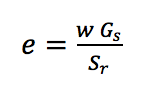(2)

which, for saturated conditions (Sr=1), simplifies to: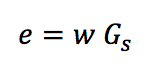(3)

A Gs value of 2.65 is typically assumed for sandy soils.

For determining w and e, another relationship needs to be developed in conjunction with equation (3). Equation (4) relates the void ratio e with the dry unit weight and specific gravity: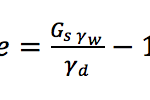(4)

Where, γw = unit weight of water (9.81 kN/m3).

For any inorganic soil, the relationship between dry unit weight γd (kN/m3), the water content and its bulk unit weight γ (kN/m3) is defined by equation (5):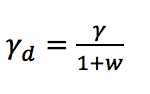(5)

By combining equations (3), (4) and (5), the following relationship is determined: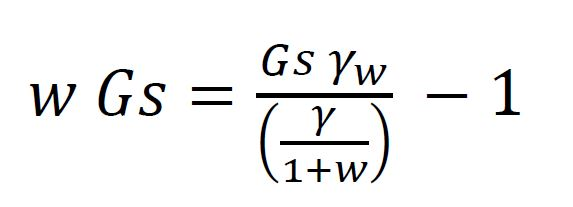(6)

In equation (6), the only unknown is the water content w. In essence, equation (6) requires that the left hand side, which expresses the full saturation of the soil, must be in equilibrium with the right hand side, which expresses the water content-bulk unit weight relationship when Gs is known. The solution for the water content at saturation (Sr=1) then is: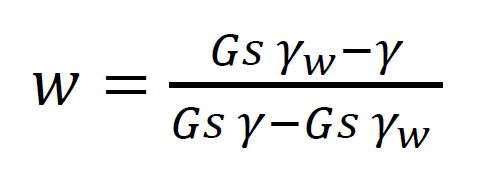(7)

Thus, for a known γ, obtained from CPT, and an appropriate assumed (or determined) value for Gs, depending on the soil type, there is a unique value for the water content w that satisfies equation (7). The void ratio and dry unit weight can then be derived from equations (3) and (5), respectively.

The porosity n can subsequently be estimated with the following relationship: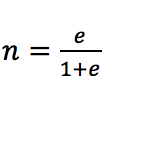(8)

The method is summarised in three steps in Figure 1.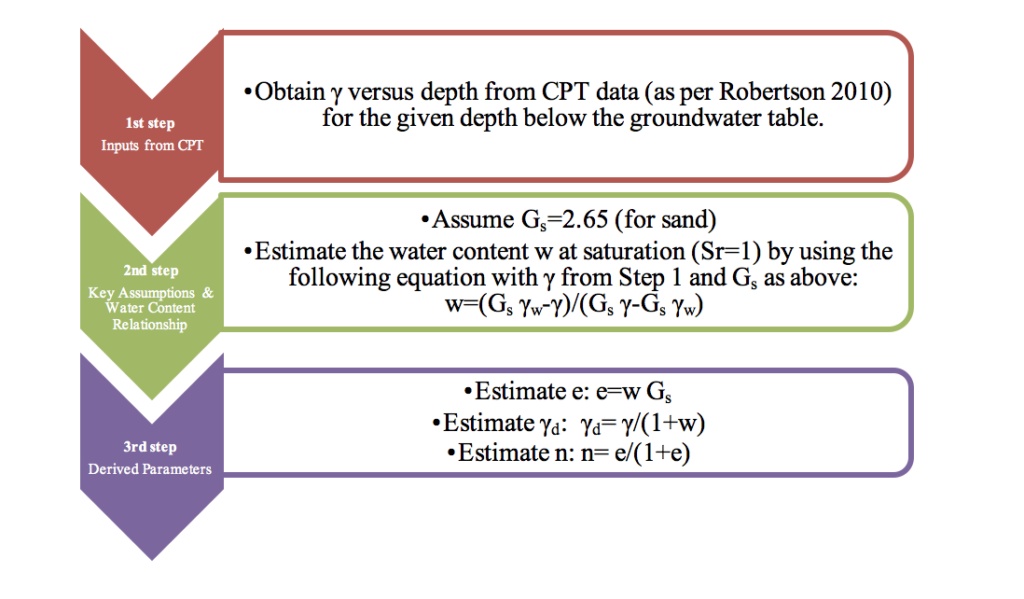Figure 1: Summary of proposed methodology for sands estimating w, e, γd and n

## 2.2 Advantages of the proposed method

The proposed method is simple and can be implemented for projects where there is a relatively shallow water table and the Gs values can be reliably assumed (or determined), particularly when laboratory tests are not included in the scope of the site investigations due to cost constraints. Where there is available budget for laboratory tests, the results from the proposed method can be checked against the laboratory results. The method can delineate against depth w, e, γd and n, and produce graphs similar to those shown in Figures 2-7, presented in Section 4 of this paper. The graphs for each of the estimated parameters begin at the depth where the groundwater surface was inferred at the given site.

# 3 TYPICAL VALUES FOR DIFFERENT TYPES OF SANDY SOIL

Typical values (Das, 2004) for water content, void ratio and dry unit weight for some cohesionless soils are presented in Table 1.

Table 1: Typical water content, void ratio and dry unit weight values for some cohesionless soils

 Type of soil Void ratio, e Water content (%), w Dry unit weight, d (kN/m3) Loose uniform sand 0.80 30 14.5 Dense uniform sand 0.45 16 18.0 Loose angular-grained silty sand 0.65 25 16.0 Dense angular-grained silty sand 0.40 15 19.0

# 4 APPLICATION FOR A CHRISTCHURCH SITE WITH SANDY SOILS

For applying the proposed methodology, a CPT was downloaded from the New Zealand Geotechnical Database. Key requirements for selecting the CPT were that:

• The site was known to have a relatively shallow groundwater table;
• The testing was undertaken on a sandy site in Christchurch, New Zealand;
• The CPT was undertaken in close proximity to a borehole, where soil samples have been taken to the laboratory to evaluate the water content;
• The number of soil samples taken at the site was adequate to compare the proposed CPT method and the laboratory testing and to draw some conclusions on the applicability of the method.

For simplicity, a specific gravity Gs of 2.65 was adopted throughout the entire depth of the CPT, as the soil profile is predominantly sands and silty sands between 4.4 to 19.5m depth. This may not be an appropriate assumption for some of the fine-grained soil layers in the upper 4.4m of the soil profile. The GWT depth was estimated to be 3m below ground level. Six graphs have been presented:

• qc versus depth
• Normalised SBTn versus depth
• Water content versus depth, showing the comparison between CPT method and laboratory testing
• Void ratio versus depth
• Bulk and dry unit weights versus depth
• Porosity versus depth

## 4.1 Borehole and sampling results

A summary of borehole and laboratory testing results are presented in Tables 2 and 3.

Table 2: Borehole summary

 Depth (m) Soil description Representative SPT N values 0.0 – 2.6 Sandy GRAVEL (no recovery between 0.2-2.0m) 22 2.6 – 4.4 SILT 4 4.4 – 5.0 Silty SAND 20 5.0 – 19.5 SAND 13-29 (Avg. = 22) 19.5 – 20.0 SILT 14

Table 3: Laboratory results summary (for sandy soils)

 Depth (m) Fines content passing 75m (%) Water content (%) 6.0 5.69 23.0 9.0 5.81 25.6 12.0 6.22 24.2 15.0 4.95 22.4

## 4.2 CPT results

CPT results and estimated parameters from the proposed methodology are presented in Figures 2-7 below.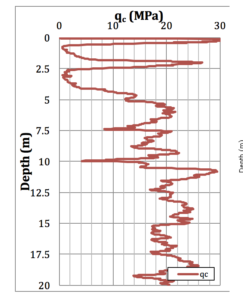Figure 2: qc plot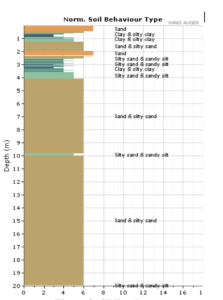Figure 3: SBTn plot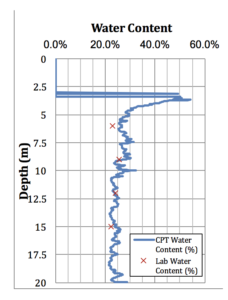Figure 4: Water content comparison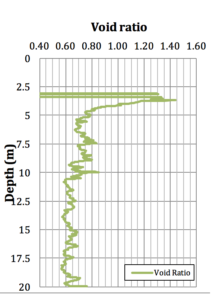Figure 5: Void ratio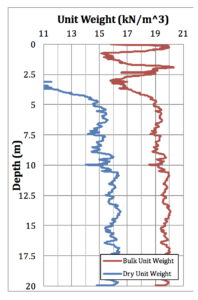Figure 6: Bulk and dry unit weights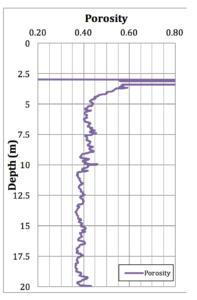Figure 7: Porosity

## 4.3 Summary

The qc (Figure 2) and SBTn (Figure 3) plots have been included to provide the reader with an understanding of the raw CPT results and soil behaviour type interpretations, which indicate that the soil predominantly consists of sands and silty sands below 4m depth. This is consistent with the borehole results.

Figure 4 shows a comparison between the CPT method for estimating water content and laboratory testing results. From this graph, it can be seen that there is a close agreement in the values returned by the two methods. This result indicates that the proposed CPT method, based on Robertson’s unit weight correlation, is accurately estimating the water content for the soil conditions encountered at this site. It can also be concluded that high quality results have been obtained from the laboratory.

Figures 5, 6 and 7 present the estimated void ratio, dry unit weight and porosity, respectively. The plots for these parameters indicate the values produced by the CPT method are consistent with typical values for the soil conditions encountered at the site, in accordance with Table 1. As these parameters have been derived based on the same assumptions as the water content, it is inferred that these estimated parameters have a similar degree of accuracy associated with them.

Table 4 shows a comparison between the laboratory water content values and the CPT estimates at the four sampling depths. An absolute difference in water content of between 0.5-3.5% (average difference of 1.8%) is considered to be an encouraging result.

Table 4: Comparison between laboratory and CPT results

 Sample Depth (m) Laboratory water content w (%) Estimated water content from CPT w (%) Absolute difference between measured and estimated w (%) 6 23.0 26.5 3.5 9 25.6 27.3 1.7 12 24.2 23.7 0.5 15 22.4 23.7 1.3

# 5 CONCLUSIONS

Based on fundamental principles of soil mechanics and the well-established published correlation for estimating bulk unit weight from CPT by Robertson (2010), and for saturated conditions with an appropriate Gs value, it is possible to estimate versus depth the:

• Water content
• Void ratio
• Dry unit weight
• Porosity

The proposed methodology can only be applied to inorganic and saturated soils. This means that the proposed methodology is applicable to coastal areas and other regions where the groundwater is typically near the ground surface. The proposed methodology was applied to a sandy site in Christchurch, New Zealand, which yielded typical results for the soil conditions encountered and showed close agreement with the laboratory testing results for the same site. It is intended to extend the methodology to estimate these soil parameters in fine-grained soils.

For projects where both CPT and laboratory testing have been undertaken, the CPT method can also be utilised as a quality control measure for the results obtained. If the underlying assumptions for the CPT method are satisfied and are sufficiently accurate for the soil conditions present at the site, there should be relatively close agreement between the CPT-estimated water content and the laboratory water content results.

The four above estimated parameters can be plotted versus depth and could be used for facilitating numerical analysis with FEM and alternative methods for estimating the following:

• Assessment of shear wave velocity
• Assessment of soil natural frequency or period
• Assessment of small-strain shear modulus
• Assessment of relative density and state of packing for cohesionless soils
• Assessment of soil permeability
• Assessment of soil groutability
• Assessment of collapse potential of soil

Further research needs to be undertaken for comparing the estimated parameters from the proposed method against the measured laboratory results for a greater number of sites.

# REFERENCES

Bowles, J.E. (1997). Foundation analysis and design, 5th Edition. New York: McGraw-Hill.

Das, B. M. (2004).Principles of foundation engineering. Brooks/Cole, Thomson Learning, U.S.A.

Robertson, P. K. and Cabal, K.L. (2010). Estimating soil unit weight from CPT. Second International Symposium on Cone Penetration Testing, Huntington Beach, CA, USA.

Robertson, P. K. and Cabal, K.L. (2015). Guide to cone penetration testing, 6th Edition. Gregg Drilling.

Save

Save

Save

Save

SaveSave

Save

SaveNZGS_Symposium_20_Barounis1 (758 KB)